Test: Number Systems, Boolean Algebra & Sequential Logic Circuits

# Test: Number Systems, Boolean Algebra & Sequential Logic Circuits

Test Description

## 20 Questions MCQ Test GATE Electrical Engineering (EE) 2023 Mock Test Series | Test: Number Systems, Boolean Algebra & Sequential Logic Circuits

Test: Number Systems, Boolean Algebra & Sequential Logic Circuits for Electrical Engineering (EE) 2023 is part of GATE Electrical Engineering (EE) 2023 Mock Test Series preparation. The Test: Number Systems, Boolean Algebra & Sequential Logic Circuits questions and answers have been prepared according to the Electrical Engineering (EE) exam syllabus.The Test: Number Systems, Boolean Algebra & Sequential Logic Circuits MCQs are made for Electrical Engineering (EE) 2023 Exam. Find important definitions, questions, notes, meanings, examples, exercises, MCQs and online tests for Test: Number Systems, Boolean Algebra & Sequential Logic Circuits below.
Solutions of Test: Number Systems, Boolean Algebra & Sequential Logic Circuits questions in English are available as part of our GATE Electrical Engineering (EE) 2023 Mock Test Series for Electrical Engineering (EE) & Test: Number Systems, Boolean Algebra & Sequential Logic Circuits solutions in Hindi for GATE Electrical Engineering (EE) 2023 Mock Test Series course. Download more important topics, notes, lectures and mock test series for Electrical Engineering (EE) Exam by signing up for free. Attempt Test: Number Systems, Boolean Algebra & Sequential Logic Circuits | 20 questions in 60 minutes | Mock test for Electrical Engineering (EE) preparation | Free important questions MCQ to study GATE Electrical Engineering (EE) 2023 Mock Test Series for Electrical Engineering (EE) Exam | Download free PDF with solutions
 1 Crore+ students have signed up on EduRev. Have you?
Test: Number Systems, Boolean Algebra & Sequential Logic Circuits - Question 1

### To perform product of maxterms Boolean function must be brought into

Test: Number Systems, Boolean Algebra & Sequential Logic Circuits - Question 2

### If (211)x = (152)8 , then the value of base x is

Detailed Solution for Test: Number Systems, Boolean Algebra & Sequential Logic Circuits - Question 2

In general, since we are comfortable dealing with Decimal system, the best way to go about under the circumstances is to convert one Number into decimal Number and then with the base x, convert another Number into Decimal Number and equate them to find the value of x.

So, (152)8 is 64*1+8*5+2, which is 106 in Decimal System.

(211)x is 2x^2+ 1*x+1 in Decimal System.

Now, we solve 2x^2+x+1 = 106

i. e. 2x^2+x-105=0

Therefore 2x^2–14x+15x-105=0

Therefore 2x(x-7)+15(x-7)=0

Therefore (x-7)(2x+15)=0

Therefore either x = 7 or x = -15/2.

Obviously x = -15/2 isnot the answer. So, x = 7 is the answer, which can be very easily verified. 2*7^2+1*7+1 = 106 which issame as (152)8.

Thus the base for 211 is 7.

Test: Number Systems, Boolean Algebra & Sequential Logic Circuits - Question 3

### 11001, 1001 and 111001 correspond to the 2’s complement representation of the following set of numbers

Detailed Solution for Test: Number Systems, Boolean Algebra & Sequential Logic Circuits - Question 3

All are 2’s complement of 7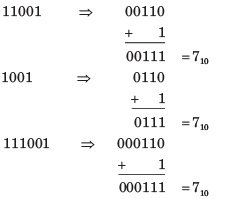Test: Number Systems, Boolean Algebra & Sequential Logic Circuits - Question 4

A signed integer has been stored in a byte using 2’s complement format. We wish to store the same integer in 16-bit word. We should copy the original byte to the less significant byte of the word and fill the more significant byte with

Detailed Solution for Test: Number Systems, Boolean Algebra & Sequential Logic Circuits - Question 4

See a example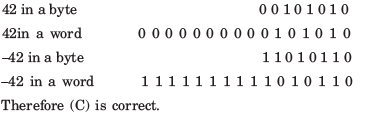Test: Number Systems, Boolean Algebra & Sequential Logic Circuits - Question 5

A computer has the following negative numbers stored in binary form as shown. The wrongly stored number is

Test: Number Systems, Boolean Algebra & Sequential Logic Circuits - Question 6

The circuit shown in fig. is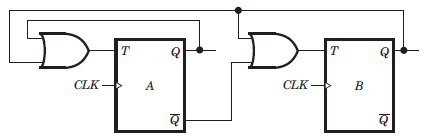Detailed Solution for Test: Number Systems, Boolean Algebra & Sequential Logic Circuits - Question 6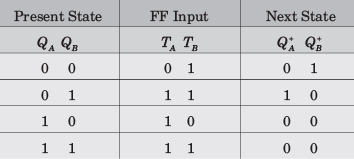From table it is clear that it is a MOD–3 counter.

Test: Number Systems, Boolean Algebra & Sequential Logic Circuits - Question 7

The counter shown in fig. is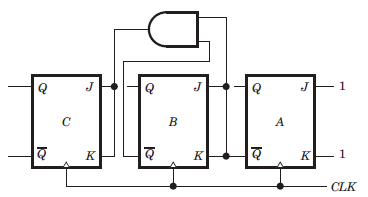Detailed Solution for Test: Number Systems, Boolean Algebra & Sequential Logic Circuits - Question 7

It is a down counter because 0 state of previous FFs change the state of next FF. You may trace the following sequence, let initial state be000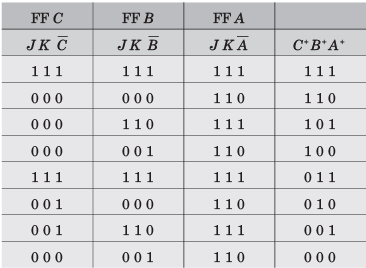Test: Number Systems, Boolean Algebra & Sequential Logic Circuits - Question 8

The counter shown in fig. counts from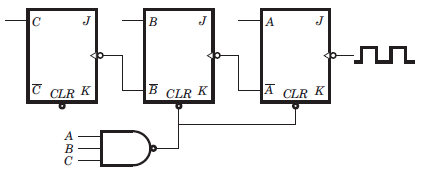Detailed Solution for Test: Number Systems, Boolean Algebra & Sequential Logic Circuits - Question 8

It is a down counter because the inverted FF output drive the clock inputs. The NAND gate will clear FFs A and B when the count tries to recycle to 111. This will produce as result of 100. Thus the counting sequence will be 100, 011, 010, 001, 000, 100 etc.

Test: Number Systems, Boolean Algebra & Sequential Logic Circuits - Question 9

The mod-number of the asynchronous counter shown in fig. is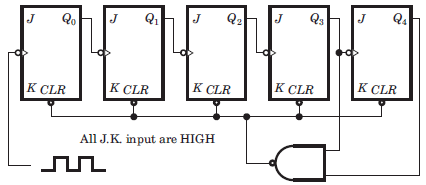Detailed Solution for Test: Number Systems, Boolean Algebra & Sequential Logic Circuits - Question 9

It is a 5 bit ripple counter. At 11000 the output of NAND gate is LOW. This will clear all FF. So it is a Mod 24 counter. Note that when 11000 occur, the CLR input is activated and all FF are immediately cleared. So it is a MOD 24 counter not MOD 25.

Test: Number Systems, Boolean Algebra & Sequential Logic Circuits - Question 10

The frequency of the pulse at z in the network shown in fig. is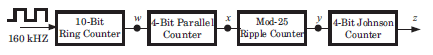Detailed Solution for Test: Number Systems, Boolean Algebra & Sequential Logic Circuits - Question 10

10-bit ring counter is a MOD–10, so it divides the 160 kHz input by 10. therefore, w = 16 kHz. The four-bit parallel counter is a MOD–16. Thus, the frequency at x = 1 kHz. The MOD–25 ripple counter produces a frequency at y = 40 Hz. (1 kHz/25 = 40 Hz).
The four-bit Johnson Counter is a MOD-8. This, the frequency at z = 5 Hz.

Test: Number Systems, Boolean Algebra & Sequential Logic Circuits - Question 11

The three-stage Johnson counter as shown in fig. is clocked at a constant frequency of fc from the starting state of Q2 Q1Q0 = 101. The frequency of output Q2 Q1Q0 will be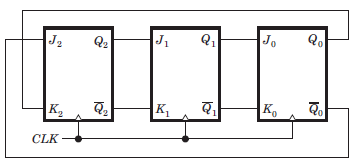Detailed Solution for Test: Number Systems, Boolean Algebra & Sequential Logic Circuits - Question 11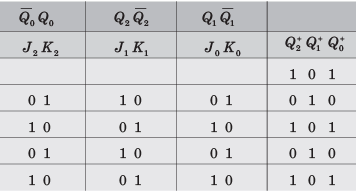We see that 1 0 1 repeat after every two cycles, hence frequency will be fc/2

Test: Number Systems, Boolean Algebra & Sequential Logic Circuits - Question 12

The counter shown in the fig. has initially Q2Q1Q0 = 000. The status of Q2 Q1Q after the first pulse is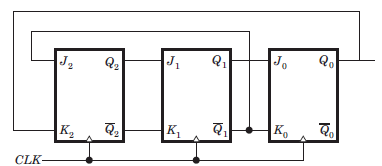Detailed Solution for Test: Number Systems, Boolean Algebra & Sequential Logic Circuits - Question 12

At first cycle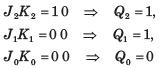Test: Number Systems, Boolean Algebra & Sequential Logic Circuits - Question 13

A 4 bit ripple counter and a 4 bit synchronous counter are made by flips flops having a propagation delay of 10 ns each. If the worst case delay in the ripple counter and the synchronous counter be R and S respectively, then

Detailed Solution for Test: Number Systems, Boolean Algebra & Sequential Logic Circuits - Question 13

In ripple counter delay 4Td = 40 ns.

The synchronous counter are clocked simultaneously, then its worst delay will be equal to 10 ns.

Test: Number Systems, Boolean Algebra & Sequential Logic Circuits - Question 14

A 4 bit modulo–6 ripple counter uses JK flip-flop. If the propagation delay of each FF is 50 ns, the maximum clock frequency that can be used is equal to

Detailed Solution for Test: Number Systems, Boolean Algebra & Sequential Logic Circuits - Question 14

4 bit uses 4 FF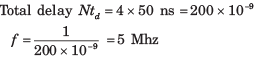Test: Number Systems, Boolean Algebra & Sequential Logic Circuits - Question 15

The initial contents of the 4-bit serial-in-parallel-out right-shift, register shown in fig. is 0 1 1 0. After three clock pulses are applied, the contents of the shift register will be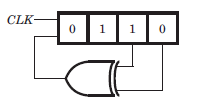Detailed Solution for Test: Number Systems, Boolean Algebra & Sequential Logic Circuits - Question 15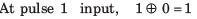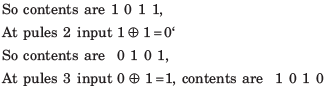Test: Number Systems, Boolean Algebra & Sequential Logic Circuits - Question 16

Consider the signed binary number A = 01010110 and B = 1110 1100 where B is the 1’s complement and MSB is the sign bit. In list-I operation is given, and in list-II resultant binary number is given.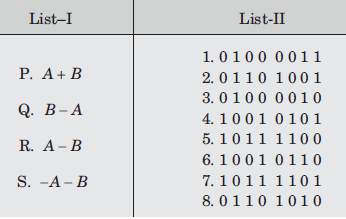The correct match is

Detailed Solution for Test: Number Systems, Boolean Algebra & Sequential Logic Circuits - Question 16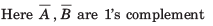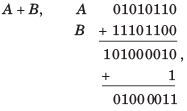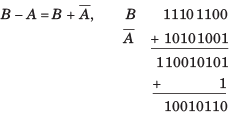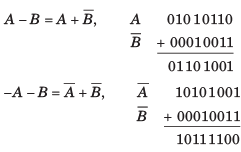Test: Number Systems, Boolean Algebra & Sequential Logic Circuits - Question 17

The simplified form of a logic function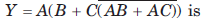Detailed Solution for Test: Number Systems, Boolean Algebra & Sequential Logic Circuits - Question 17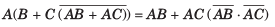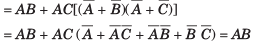Test: Number Systems, Boolean Algebra & Sequential Logic Circuits - Question 18

If the decimal number is a fraction then its binary equivalent is obtained by ________ the number continuously by 2.

Detailed Solution for Test: Number Systems, Boolean Algebra & Sequential Logic Circuits - Question 18

On multiplying the decimal number continuously by 2, the binary equivalent is obtained.

Test: Number Systems, Boolean Algebra & Sequential Logic Circuits - Question 19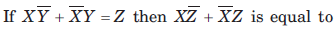Detailed Solution for Test: Number Systems, Boolean Algebra & Sequential Logic Circuits - Question 19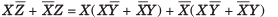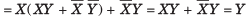Test: Number Systems, Boolean Algebra & Sequential Logic Circuits - Question 20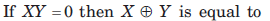Detailed Solution for Test: Number Systems, Boolean Algebra & Sequential Logic Circuits - Question 20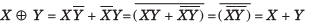## GATE Electrical Engineering (EE) 2023 Mock Test Series

21 docs|271 tests
 Use Code STAYHOME200 and get INR 200 additional OFF Use Coupon Code
Information about Test: Number Systems, Boolean Algebra & Sequential Logic Circuits Page
In this test you can find the Exam questions for Test: Number Systems, Boolean Algebra & Sequential Logic Circuits solved & explained in the simplest way possible. Besides giving Questions and answers for Test: Number Systems, Boolean Algebra & Sequential Logic Circuits, EduRev gives you an ample number of Online tests for practice

## GATE Electrical Engineering (EE) 2023 Mock Test Series

21 docs|271 tests